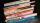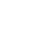# Fractions + greatest common divisor (GCD) - math problems

#### Number of problems found: 6

• LCD 2The least common denominator of 2/5, 1/2, and 3/4
• SelfA math text book is 2 2/9 inches thick. how many of these books will fit on a 120-inch self?
• Reminder and quotientThere are given the number C = 281, D = 201. Find the highest natural number S so that the C:S, D:S are with the remainder of 1,
• Ornamental shrubsChildren committed to plant 240 ornamental shrubs. Their commitment however exceeded by 48 shrubs. Write ratio of actually planted shrubs and commitment by lowest possible integers a/b.
• A largeA large gear will be used to turn a smaller gear. The large gear will make 75 revolutions per minute. The smaller gear must make 384 revolutions per minute. Find the smallest number of teeth each gear could have. [Hint: Use either GCF or LCM. ]
• Profitable companyThree businessman decide to open up their own company. They agree to distribute the yearly profits made in the same ratio as their initial investments. They invest R 50 000, R 75 000 and R25 000, respectively. The profit made by the company in the first y

We apologize, but in this category are not a lot of examples.
Do you have an interesting mathematical word problem that you can't solve it? Submit a math problem, and we can try to solve it.

We will send a solution to your e-mail address. Solved examples are also published here. Please enter the e-mail correctly and check whether you don't have a full mailbox.

Please do not submit problems from current active competitions such as Mathematical Olympiad, correspondence seminars etc...

Do you want to calculate greatest common divisor two or more numbers? Need help calculate sum, simplify or multiply fractions? Try our fraction calculator. Fraction Word Problems. Greatest common divisor (GCD) - math word problems.